International
Tables for
Crystallography
Volume B
Reciprocal space
Edited by U. Shmueli

International Tables for Crystallography (2010). Vol. B, ch. 2.5, pp. 367-368   | 1 | 2 |

## Section 2.5.6.2. 3D reconstruction in the general case

B. K. Vainshteinc and P. A. Penczekg

#### 2.5.6.2. 3D reconstruction in the general case

| top | pdf |

In the general case of the 3D reconstruction of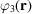from projections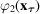, the projection vectoroccupies arbitrary positions on the projection sphere (Fig. 2.5.6.2). First, let us consider the case of a 2D function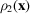and its ray transform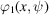. We introduce an operation of backprojection b, which is stretching along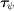each 1D projection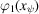(Fig. 2.5.6.3). When the result is integrated over the full angular range of projections, we obtain the projection synthesis defined as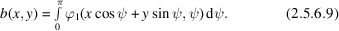However, the backprojection operator is not the inverse of a 2D ray transform, as the resulting image b is blurred by the point-spread function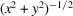(Vainshtein, 1971):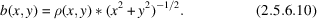By noting that the Fourier transform ofis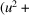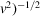and by using the convolution theorem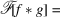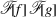, we obtain the backprojection-filtering' inversion formula: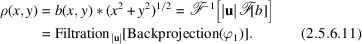The more commonly used filtered-backprojection' inversion is based on the 2D version of the central section theorem (2.5.6.8):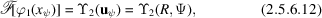where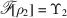. With this in mind,can be related to its ray transform by evaluating the Fourier transform of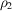in polar coordinates: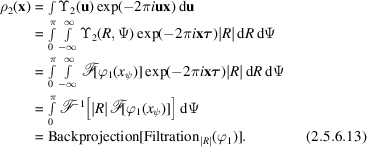In three dimensions, the backprojection stretches each 2D projection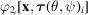along the projection direction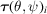. A 3D synthesis is the integral over the hemisphere (Fig. 2.5.6.2)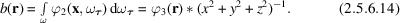Thus, in three dimensions the image b obtained using the backprojection operator is blurred by the point-spread function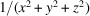(Vainshtein, 1971). It is possible to derive inversion formulae analogous to (2.5.6.11)and (2.5.6.13).Figure 2.5.6.3 | top | pdf |(a) Formation of a backprojection function; (b) projection synthesis (2.5.6.9)is a superposition of these functions.

The inversion formulae demonstrate that it is possible to invert the ray transform for continuous functions and for a uniform distribution of projections. In electron microscopy, the projections are never distributed uniformly in three dimensions. Indeed, a uniform distribution is not even desirable, as only certain subsets of projection directions are required for the successful inversion of a 3D ray transform, as follows from the central section theorem (2.5.6.8). In practice, we always deal with sampled data and with discrete, random and nonuniform distributions of projection directions. Therefore, the inversion formulae can be considered only as a starting point for the development of the numerical (and practical!) reconstruction algorithms. According to (2.5.6.10)and (2.5.6.14), a simple backprojection step results in reconstruction that corresponds to a convolution of the original function with a point-spread function that depends only on the distribution of projections, but not on the structure itself. Taking into account the linearity of the backprojection operation, one has to conclude that for any practically encountered distribution of projections it should be possible to derive the corresponding point-spread function and then, using either deconvolution or Fourier filtration (with a `weighting function'), arrive at a good approximation of the structure. This observation forms the basis of the weighted backprojection algorithm (Section 2.5.6.5). Similarly, the central section theorem gives rise to direct Fourier inversion algorithms (Section 2.5.6.6). Nevertheless, since the data are discrete, the most straightforward methodology is to discretize and approach the reconstruction problem as an algebraic problem (Section 2.5.6.4).

### References

Vainshtein, B. K. (1971). Finding the structure of objects from projections. Sov. Phys. Crystallogr. 15, 781–787.